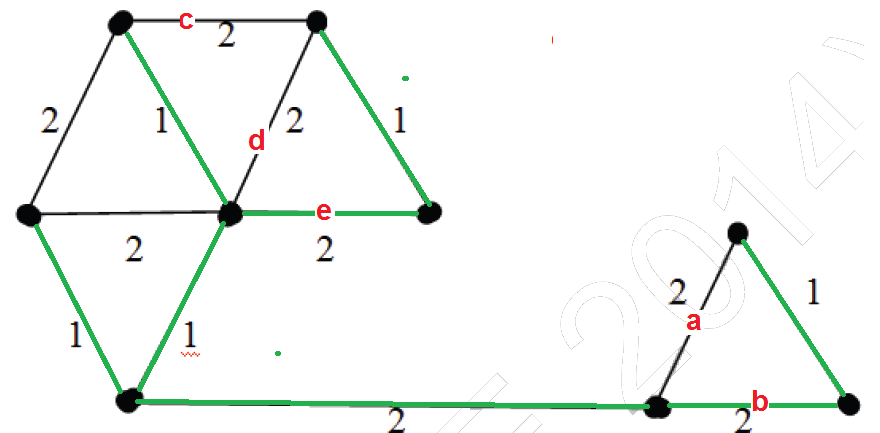4.4k views

The number of distinct minimum spanning trees for the weighted graph below is _____| 4.4k views
+3

One can use Kirchof's theorem to get distinct minimum spanning trees .....

+7
I guess, Kirchoff's theorem is used to find total no. of spanning Trees, not the MSTs?
0

@vishalshrm539

And it should be unweighted graph too?

$6$ is the answer.

$2\times3=6$ possibilitiesby Veteran (431k points)
edited
0
Sir,could you explain? actually i want to know if there is any shortcut for finding total number of minimum spanning trees of a Graph.
The steps i have taken to answer this question
1.I used krushkal's algorithm for finding all the minimum spanning trees.
2..Then i counted all the total no of minimum spanning trees.
But that is a lengthy process & takes some time..So i want to know.. is there any other method for fining it easily? Waiting for your Reply :)
+7
Consider any cycle in a MST- the maximum weighted edge won't be in MST- if there are multiple edges of same max. weight- we get multiple MSTs. This property can be used, though not straight forward.
0
sir, is there any formula to find no of spanning tree?
0
nice explanation @Arjun sir
0
I Think that the answer might be 9! we have 3 possibilities on the left and 3 on the right.
+1 vote
3*2=6

Delete all 2 edges and try to form spanning tree but you cannt

Therefore there there are 3 choices for

Upper right section and 2 for bottom triangle
by (457 points)

Below diagram shows a minimum spanning tree. Highlighted (in green) are the edges picked to make the MST.In the right side of MST, we could either pick edge ‘a’ or ‘b’. In the left side, we could either pick ‘c’ or ‘d’ or ‘e’ in MST.

There are 2 options for one edge to be picked and 3 options for another edge to be picked. Therefore, total 2*3 possible MSTs.

https://www.geeksforgeeks.org/gate-gate-cs-2014-set-2-question-62/

ago by Loyal (5.8k points)